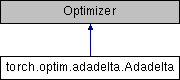Caffe2 - Python API A deep learning, cross platform ML framework## Public Member Functions

def __init__ (self, params, lr=1.0, rho=0.9, eps=1e-6, weight_decay=0)

def step (self, closure=None)

## Detailed Description

```Implements Adadelta algorithm.

Arguments:
params (iterable): iterable of parameters to optimize or dicts defining
parameter groups
rho (float, optional): coefficient used for computing a running average
eps (float, optional): term added to the denominator to improve
numerical stability (default: 1e-6)
lr (float, optional): coefficient that scale delta before it is applied
to the parameters (default: 1.0)
weight_decay (float, optional): weight decay (L2 penalty) (default: 0)

__ https://arxiv.org/abs/1212.5701
```

## Member Function Documentation

 def torch.optim.adadelta.Adadelta.step ( self, closure = `None` )
```Performs a single optimization step.

Arguments:
closure (callable, optional): A closure that reevaluates the model
and returns the loss.
```